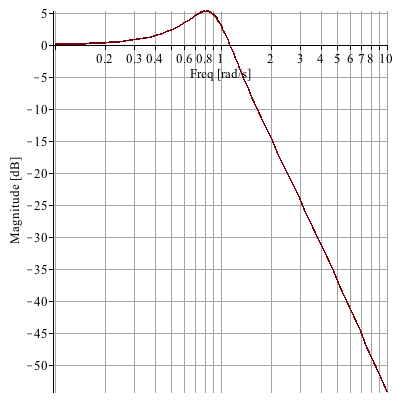New Features in Maple 17: Control DesignControl design tools in Maple have been enhanced to become even more flexible.  Improvements to the DynamicSystems package include:

• The FrequencyResponse routine handles differential equations with input derivatives.
• All models now accept linear, non-differential systems.
• A new frequencies option for all frequency-based plots enables you to  specify the precise frequencies at which expressions are evaluated.
• The Grammians command has been extended to work with discrete systems.
• Nichols plots are now accessible from the context-sensitive menu.

Example

 >Assign a differential system with derivatives in the input.

 >>Plot the magnitude of the response vs frequency, adding circles at selected frequencies. This is done by generating and combining two plots.

 >Example

A Nichols plot is useful for quickly estimating the closed-loop response of system with unity-feedback, given its open-loop transfer function. For example, let the open-loop transfer function be:

 >By default a Nichols plot includes constant-phase and constant-magnitude contour plots of a closed-loop system.  From the graph below, the peak closed-loop response is about 5 dB, at 0.8 rad/s, because that is the highest constant-magnitude contour that it touches (estimating).

 >Here we plot the actual closed-loop response and confirm that the maximum gain is approximately 5 dB, at 0.8 rad/s.

 >>